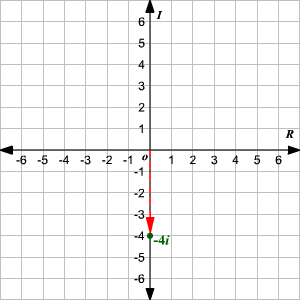# Complex Numbers

complex number  is a number of the form, whereandare real numbers andis the imaginary unit , the square root of.

In a complex number,is called the "real part" ofandis called the "imaginary part." If, the complex number is a real number; if, then the complex number is "purely imaginary."

We can graph a complex number on the Cartesian plane , using the horizontal axis as the real axis and the vertical axis as the imaginary axis. When we use the Cartesian plane this way, we call it the complex plane .

The complex numbercan be plotted as the ordered pairon the complex plane.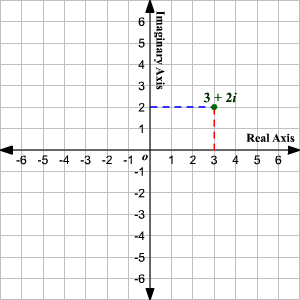The absolute value or modulus of a complex numbercan be interpreted as the distance of the pointfrom the origin on a complex plane.

Using the Distance Formula,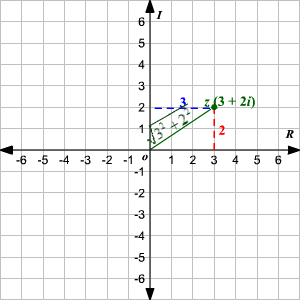Example 1:

Plot the numberon a complex plane.

The real part of the complex number isand the imaginary part is.

Start at the origin. Moveunits to the left on the real axis to reach the point. Now, moveunits upward to reach the point.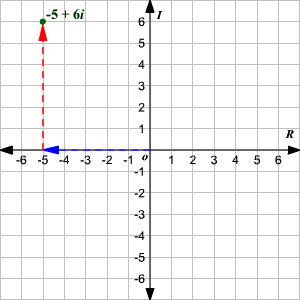If the real part of a complex number is zero, the number lies on the imaginary axis. Similarly, if the imaginary part of a complex number is zero, the number lies on the real axis.

Example 2:

Plot the numberon the complex plane.

The real part of the complex number isand the imaginary part is. So, the number will lie on the real axis.

Start at the origin. Moveunits to the right on the real axis to reach the point.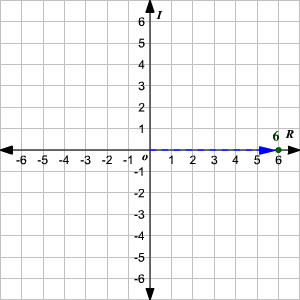Example 3:

Plot the numberon the complex plane.

The real part of the complex numberis zero and the imaginary part is.

Start at the origin. Moveunits down along the imaginary axis to reach the point.